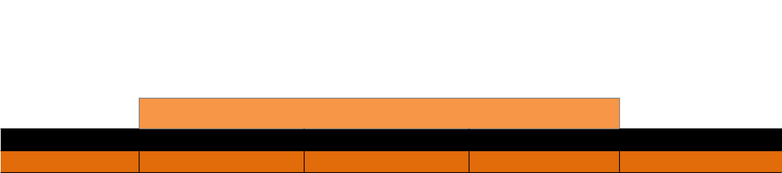Class Notes (1,100,000)
CA (620,000)
U of G (30,000)
ACCT (200)
Lecture 15

# ACCT 2230 Lecture Notes - Lecture 15: Standard Deviation, Quartile, Interquartile Range

Department
Accounting
Course Code
ACCT 2230
Professor
Steven J Maynard
Lecture
15

This preview shows half of the first page. to view the full 3 pages of the document.Assignment #2
Part 3: Percentile
Percentiles
5th Percentile Quartile 1 (25th) Quartile 2 (Median) Quartile 3 (75th) 95th Percentile
\$810,000.00 \$3,000,000.00 \$4,100,000.00 5,703,125.00 \$7,500,000.00
When viewing the percentiles, the first number that we look at is the 2nd quartile
or the median value. This number is the middle value of your data that separates the top
50% from the bottom 50%. From our table we are able to see that the median value (or
salary) is \$4,100,000.00. This value was located at the 50th value when our Y-variable
was ordered smallest to largest. When looking at this median we can see that it does
almost split both the 5th and the 95th percentiles as well as the first and second quartiles
in half. This information shows us the median value is not majorly skewed by any
extreme values at either end.
The gap between the first and second and the second and third quartiles is very
similar in size (the gap between the second and third being slightly larger). This shows
us that when you move between the second and third quartile the salaries will increase
slightly faster than when you move between the first and second quartiles or there may
be an extreme value at the higher end. When calculating the interquartile range
(5,703,125 – 3,000,000 = 2,703,125) we see that there is no major fluctuation in the
mid-section of our data.
The 5th and 95th percentiles are both extreme points but not extreme enough to
provide a skew to the data. If viewing the 100th percentile which we were able to see in
our table of frequencies we can see that it may provide a slight skew because it is the
most extreme point. However, this is not able to be seen by only viewing the 5th and 95th
percentiles. The 95th percentile is again a slight bit further away from the median than
the 5th percentile which tells us that we most likely extreme value at the higher end that
have more impact on the skew of the data then at the lower end.